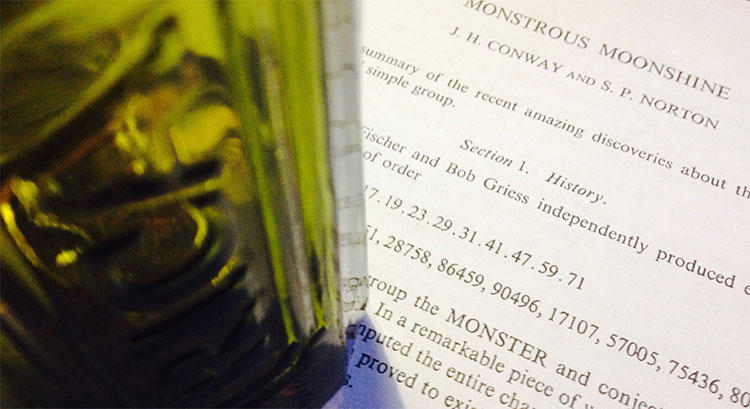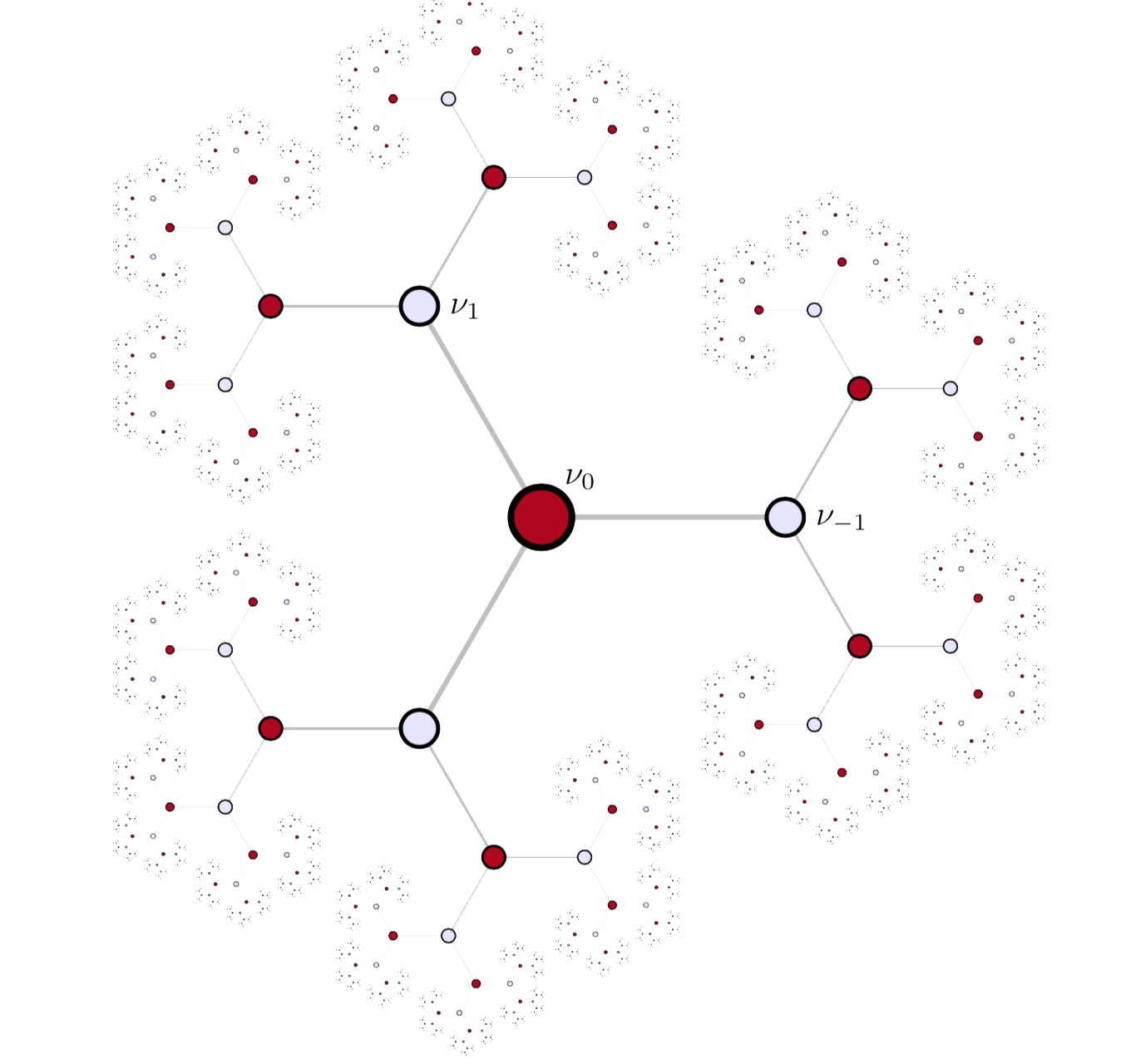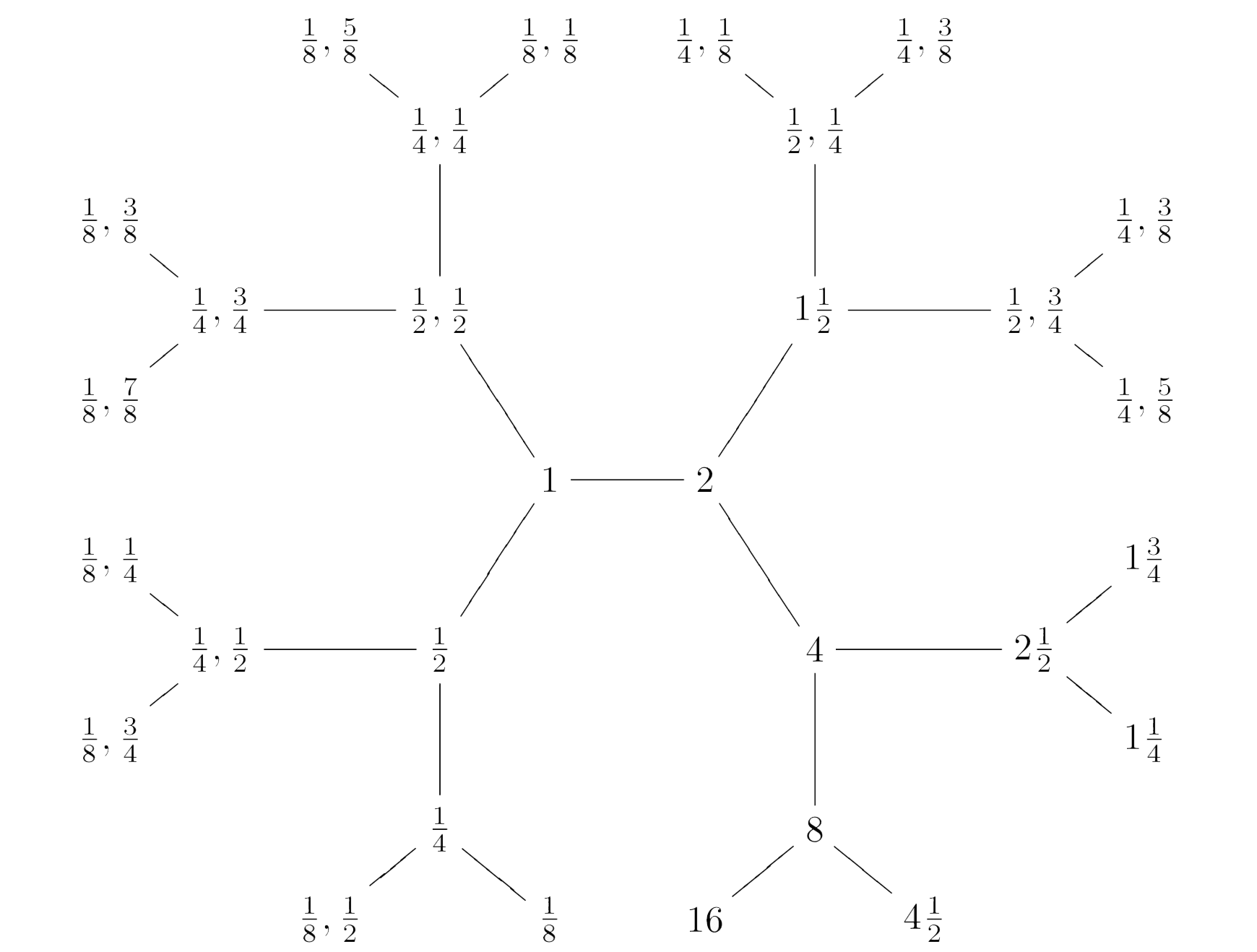# Tag: TitsAt a seminar at the College de France in 1975, Tits wrote down the order of the monster group

$\# \mathbb{M} = 2^{46}.3^{20}.5^9.7^6.11^2.13^3.17·19·23·29·31·41·47·59·71$

Andrew Ogg, who attended the talk, noticed that the prime divisors are precisely the primes $p$ for which the characteristic $p$ super-singular $j$-invariants are all defined over $\mathbb{F}_p$.

Here’s Ogg’s paper on this: Automorphismes de courbes modulaires, Séminaire Delange-Pisot-Poitou. Théorie des nombres, tome 16, no 1 (1974-1975).

Ogg offered a bottle of Jack Daniels for an explanation of this coincidence.

Even Richard Borcherds didn’t claim the bottle of Jack Daniels, though his proof of the monstrous moonshine conjecture is believed to be the best explanation, at present.

A few years ago, John Duncan and Ken Ono posted a paper “The Jack Daniels Problem”, in which they prove that monstrous moonshine implies that if $p$ is not one of Ogg’s primes it cannot be a divisor of $\# \mathbb{M}$. However, the other implication remains mysterious.

Duncan and Ono say:

“This discussion does not prove that every $p ∈ \text{Ogg}$ divides $\# \mathbb{M}$. It merely explains how the first principles of moonshine suggest this implication. Monstrous moonshine is the proof. Does this then provide a completely satisfactory solution to Ogg’s problem? Maybe or maybe not. Perhaps someone will one day furnish a map from the characteristic $p$ supersingular $j$-invariants to elements of order $p$ where the group structure of $\mathbb{M}$ is apparent.”

I don’t know whether they claimed the bottle, anyway.

But then, what is the non-commutative Jack Daniels Problem?

A footnote on the first page of Conway and Norton’s ‘Monstrous Moonshine’ paper says:

“Very recently, A. Pizer has shown these primes are the only ones that satisfy a certain conjecture of Hecke from 1936 relating modular forms of weight $2$ to quaternion algebra theta-series.”

Pizer’s paper is “A note on a conjecture of Hecke”.

Maybe there’s a connection between monstrous moonshine and the arithmetic of integral quaternion algebras. Some hints:

The commutation relations in the Big Picture are reminiscent of the meta-commutation relations for Hurwitz quaternions, originally due to Conway in his booklet on Quaternions and Octonions.

The fact that the $p$-tree in the Big Picture has valency $p+1$ comes from the fact that the Brauer-Severi of $M_2(\mathbb{F}_p)$ is $\mathbb{P}^1_{\mathbb{F}_p}$. In fact, the Big Picture should be related to the Brauer-Severi scheme of $M_2(\mathbb{Z})$.

Then, there’s Jorge Plazas claiming that Connes-Marcolli’s $GL_2$-system might be related to moonshine.

One of the first things I’ll do when I return is to run to the library and get our copy of Shimura’s ‘Introduction to the arithmetic theory of automorphic functions’.

Btw. the bottle in the title image is not a Jack Daniels but the remains of a bottle of Ricard, because I’m still in the French mountains.

Conway’s Big Picture consists of all pairs of rational numbers $M,\frac{g}{h}$ with $M > 0$ and $0 \leq \frac{g}{h} < 1$ with $(g,h)=1$. Recall from last time that $M,\frac{g}{h}$ stands for the lattice
$\mathbb{Z} (M \vec{e}_1 + \frac{g}{h} \vec{e}_2) \oplus \mathbb{Z} \vec{e}_2 \subset \mathbb{Q}^2$
and we associate to it the rational $2 \times 2$ matrix
$\alpha_{M,\frac{g}{h}} = \begin{bmatrix} M & \frac{g}{h} \\ 0 & 1 \end{bmatrix}$

If $M$ is a natural number we write $M \frac{g}{h}$ and call the corresponding lattice number-like, if $g=0$ we drop the zero and write $M$.

The Big Picture carries a wealth of structures. Today, we will see that it can be factored as the product of Bruhat-Tits buildings for $GL_2(\mathbb{Q}_p)$, over all prime numbers $p$.

Here’s the factor-building for $p=2$, which is a $3$-valent tree:To see this, define the distance between lattices to be
$d(M,\frac{g}{h}~|~N,\frac{i}{j}) = log~Det(q(\alpha_{M,\frac{g}{h}}.\alpha_{N,\frac{i}{j}}^{-1}))$
where $q$ is the smallest strictly positive rational number such that $q(\alpha_{M,\frac{g}{h}}.\alpha_{N,\frac{i}{j}}^{-1}) \in GL_2(\mathbb{Z})$.

We turn the Big Picture into a (coloured) graph by drawing an edge (of colour $p$, for $p$ a prime number) between any two lattices distanced by $log(p)$.

$\xymatrix{M,\frac{g}{h} \ar@[red]@{-}[rr]|p & & N,\frac{i}{j}} \qquad~\text{iff}~\qquad d(M,\frac{g}{h}~|~N,\frac{i}{j})=log(p)$

The $p$-coloured subgraph is $p+1$-valent.

The $p$-neighbours of the lattice $1 = \mathbb{Z} \vec{e}_1 \oplus \mathbb{Z} \vec{e}_2$ are precisely these $p+1$ lattices:

$p \qquad \text{and} \qquad \frac{1}{p},\frac{k}{p} \qquad \text{for} \qquad 0 \leq k < p$ And, multiplying the corresponding matrices with $\alpha_{M,\frac{g}{h}}$ tells us that the $p$-neighbours of $M,\frac{g}{h}$ are then these $p+1$ lattices: $pM,\frac{pg}{h}~mod~1 \qquad \text{and} \qquad \frac{M}{p},\frac{1}{p}(\frac{g}{h}+k)~mod~1 \qquad \text{for} \qquad 0 \leq k < p$ Here's part of the $2$-coloured neighbourhood of $1$To check that the $p$-coloured subgraph is indeed the Bruhat-Tits building of $GL_2(\mathbb{Q}_p)$ it remains to see that it is a tree.

For this it is best to introduce $p+1$ operators on lattices

$p \ast \qquad \text{and} \qquad \frac{k}{p} \ast \qquad \text{for} \qquad 0 \leq k < p$ defined by left-multiplying $\alpha_{M,\frac{g}{h}}$ by the matrices $\begin{bmatrix} p & 0 \\ 0 & 1 \end{bmatrix} \qquad \text{and} \qquad \begin{bmatrix} \frac{1}{p} & \frac{k}{p} \\ 0 & 1 \end{bmatrix} \qquad \text{for} \qquad 0 \leq k < p$ The lattice $p \ast M,\frac{g}{h}$ lies closer to $1$ than $M,\frac{g}{h}$ (unless $M,\frac{g}{h}=M$ is a number) whereas the lattices $\frac{k}{p} \ast M,\frac{g}{h}$ lie further, so it suffices to show that the $p$ operators $\frac{0}{p} \ast,~\frac{1}{p} \ast,~\dots~,\frac{p-1}{p} \ast$ form a free non-commutative monoid.
This follows from the fact that the operator
$(\frac{k_n}{p} \ast) \circ \dots \circ (\frac{k_2}{p} \ast) \circ (\frac{k_1}{p} \ast)$
is given by left-multiplication with the matrix
$\begin{bmatrix} \frac{1}{p^n} & \frac{k_1}{p^n}+\frac{k_2}{p^{n-1}}+\dots+\frac{k_n}{p} \\ 0 & 1 \end{bmatrix}$
which determines the order in which the $k_i$ occur.

A lattice at distance $n log(p)$ from $1$ can be uniquely written as
$(\frac{k_{n-l}}{p} \ast) \circ \dots \circ (\frac{k_{l+1}}{p} \ast) \circ (p^l \ast) 1$
which gives us the unique path to it from $1$.

The Big Picture itself is then the product of these Bruhat-Tits trees over all prime numbers $p$. Decomposing the distance from $M,\frac{g}{h}$ to $1$ as
$d(M,\frac{g}{h}~|~1) = n_1 log(p_1) + \dots + n_k log(p_k)$
will then allow us to find minimal paths from $1$ to $M,\frac{g}{h}$.

But we should be careful in drawing $2$-dimensional cells (or higher dimensional ones) in this ‘product’ of trees as the operators
$\frac{k}{p} \ast \qquad \text{and} \qquad \frac{l}{q} \ast$
for different primes $p$ and $q$ do not commute, in general. The composition
$(\frac{k}{p} \ast) \circ (\frac{l}{q} \ast) \qquad \text{with matrix} \qquad \begin{bmatrix} \frac{1}{pq} & \frac{kq+l}{pq} \\ 0 & 1 \end{bmatrix}$
has as numerator in the upper-right corner $0 \leq kq + l < pq$ and this number can be uniquely(!) written as $kq+l = up+v \qquad \text{with} \qquad 0 \leq u < q,~0 \leq v < p$ That is, there are unique operators $\frac{u}{q} \ast$ and $\frac{v}{p} \ast$ such that $(\frac{k}{p} \ast) \circ (\frac{l}{q} \ast) = (\frac{u}{q} \ast) \circ (\frac{v}{p} \ast)$ which determine the $2$-cells $\xymatrix{ \bullet \ar@[blue]@{-}[rr]^{\frac{u}{q} \ast} \ar@[red]@{-}[dd]_{\frac{v}{p} \ast} & & \bullet \ar@[red]@{-}[dd]^{\frac{k}{p} \ast} \\ & & \\ \bullet \ar@[blue]@{-}[rr]_{\frac{l}{q} \ast} & & \bullet}$ These give us the commutation relations between the free monoids of operators corresponding to different primes.
For the primes $2$ and $3$, relevant in the description of the Moonshine Picture, the commutation relations are

$(\frac{0}{2} \ast) \circ (\frac{0}{3} \ast) = (\frac{0}{3} \ast) \circ (\frac{0}{2} \ast), \quad (\frac{0}{2} \ast) \circ (\frac{1}{3} \ast) = (\frac{0}{3} \ast) \circ (\frac{1}{2} \ast), \quad (\frac{0}{2} \ast) \circ (\frac{2}{3} \ast) = (\frac{1}{3} \ast) \circ (\frac{0}{2} \ast)$

$(\frac{1}{2} \ast) \circ (\frac{0}{3} \ast) = (\frac{1}{3} \ast) \circ (\frac{1}{2} \ast), \quad (\frac{1}{2} \ast) \circ (\frac{1}{3} \ast) = (\frac{2}{3} \ast) \circ (\frac{0}{2} \ast), \quad (\frac{1}{2} \ast) \circ (\frac{2}{3} \ast) = (\frac{2}{3} \ast) \circ (\frac{1}{2} \ast)$